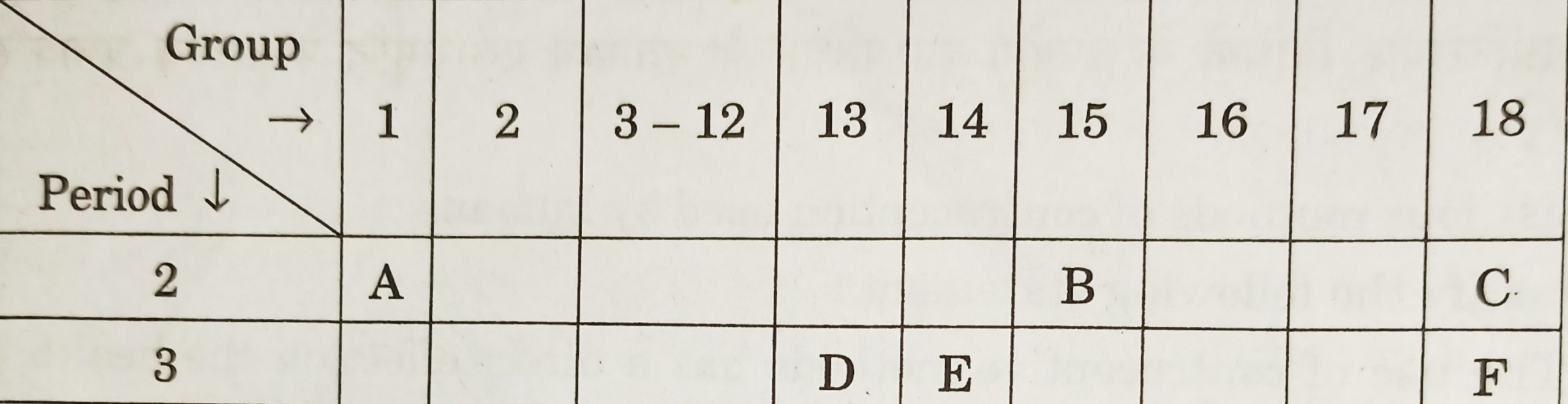# Class 10 – Periodic Classification Of Elements – Previous Years Questions

Periodic Classification Of Elements – Previous Years Questions

1. Write the atomic numbers of two elements ‘X’ and ‘Y’ having electronic configurations 2, 8, 2 and 2, 8, 6 respectively. [CBSE 2014] [1 Marks]
2. State the common characteristics of the following elements:
Boron, Silicon, Germanium and Arsenic [CBSE 2020] [1 Marks]
3. An element X with atomic number 12 forms a compound with element Y with atomic number 17. The formula of compound formed is
1. XY
2. XY2
3. X2Y
4. X2Y3 [CBSE 2020] [1 Mark]
4. An element X is forming acidic oxide. Its most probable position in the modern periodic table is
1. Group 1 and period 3
2. Group 16 and Period 3
3. Group 17 and Period 3
4. Group 2 and Period 3 [CBSE 2020] [1 Mark]
5. State the periodic law on which the Modern Periodic Table is based. [CBSE 2020] [1 Marks]
6. Study the following table in which the positions of six elements A, B, C, D, E and F are shown as they are in the modern periodic table:On the basis of the above table, answer the following questions :
1. Name the element which forms only covalent compounds.
2. Name the element which is a metal with valency three.
3. Name the element which is a non-metal with valency three.
4. Out of D and E, Which is bigger in size and why ?
5. Write the common name for the family to which the elements C and F belong. [CBSE 2014] [3 Marks]
7. What is meant by ‘group’ in the modern periodic table? How do the following change on moving from top to bottom in a group?
1. Number of valence electrons
2. Number of occupied shells
3. Size of atoms
4. Metallic characters of elements
5. Effective nuclear charge experienced by valence electrons [CBSE 2014] [3 Marks]
8. An element ‘X’ belongs to third period and group 16 of the Modern Periodic Table.
1. Determine the number of valence electrons and the valency of ‘X’.
2. Molecular formula of the compound when ‘X’ reacts with hydrogen and write its electron dot structure.
3. Name the element ‘X’ and state weather it is metallic or non-metallic. [CBSE 2016] [3 Marks]
9. Three elements ‘X’ ‘Y’ and ‘Z’have atomic numbers 7, 8 and 9 respectively .
1. State the positions (Group number and period number both) in the Modern Periodic Table.
2. Arrange these elements in the decreasing order of their atomic radii.
3. Write the formula of compound formed when ‘X’ combines with ‘Z’. [CBSE 2016] [3 Marks]
10. .
1. Name the element with atomic number 12.
2. In which group it is placed?
3. In which period it is positioned?
4. Write down its electronic configuration. [CBSE 2017] [3 Marks]
11. “Atomic number of an element is considered to be more appropriate parameter than its atomic mass for a chemist.” Take the example of the element X (atomic number 13) to justify this statement. [CBSE 2019] [3 Marks]
12. .
1. How is valency of an element determined from the electronic configuration of its atom?
2. Determine the valency of an element X whose atomic number is 15. [Cbse 2019] 3 Marks]
13. From the elements Li, K, Mg, C, Al, S identify the
1. elements belonging to the same group.
2. element which has the tendency to lose two electrons.
3. element which prefers sharing of electrons to complete its octet.
4. most metallic element.
5. element that forms acidic oxide.
6. element that belongs to group 13. [CBSE 2020] [3 Marks]
14. .
1. List any two distinguishing features between Mendeleev’s Periodic Table and the Modern Periodic Table.
2. With the help of an example, explain Dobereiner’s Triads.
3. State modern periodic law. [CBSE 2020] [3 Marks]
15. The formulae of oxides of two elements X and Y are XO and Y2O3 respectively.
1. Find the valencies of X and Y.
2. Identify the groups in which they would be placed in the modern periodic table.
3. Name one more element belonging to each of these groups. [CBSE 2017] [5 Marks]
16. .
1. The modern periodic table has been evolved through the early attempts of Dobereiner, Newland and Mendeleev. List one advantage and one limitation of all the three attempts.
2. Name the scientist who first of all showed that atomic number of an element is a more fundamental property than its atomic mass.
3. State modern periodic law. [CBSE 2018] [5 Marks]